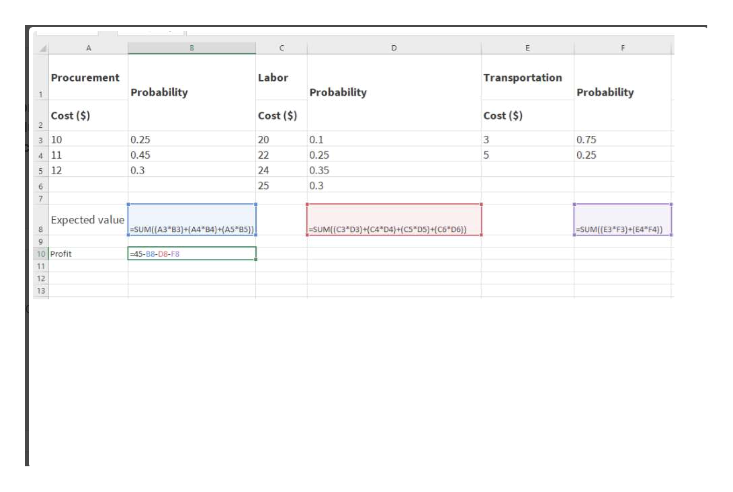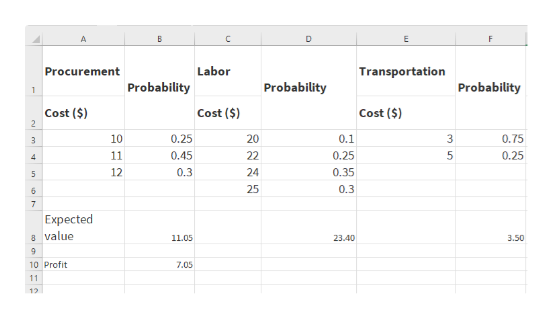# (All answers were generated using 1,000 trials and native Excel functionality.)The management of Brinkley Corporation is interested in using simulation to estimate the profit per unit for a new product. The selling price for the product will be \$45 per unit. Probability distributions for the purchase cost, the labor cost, and the transportation cost are estimated as follows: ProcurementCost (\$)ProbabilityLaborCost (\$)ProbabilityTransportationCost (\$)Probability100.25200.1030.75110.45220.2550.25120.30240.35    250.30    (a)Construct a simulation model to estimate the average profit per unit. What is a 95% confidence interval around this average? Round your answers to two decimal places. Lower Bound: \$ Upper Bound: \$

Question
86 views

(All answers were generated using 1,000 trials and native Excel functionality.)

The management of Brinkley Corporation is interested in using simulation to estimate the profit per unit for a new product. The selling price for the product will be \$45 per unit. Probability distributions for the purchase cost, the labor cost, and the transportation cost are estimated as follows:

 ProcurementCost (\$) Probability LaborCost (\$) Probability TransportationCost (\$) Probability 10 0.25 20 0.10 3 0.75 11 0.45 22 0.25 5 0.25 12 0.30 24 0.35 25 0.30

 (a) Construct a simulation model to estimate the average profit per unit. What is a 95% confidence interval around this average? Round your answers to two decimal places. Lower Bound: \$ Upper Bound: \$
check_circle

star
star
star
star
star
1 Rating
Step 1

Expected value of profit can be calculated by using formula: Selling price - Expected value of Procurement cost - Expected value of Labour cost - Expected value of Transportation costhelp_outlineImage TranscriptioncloseLabor Transportation Procurement Probability Probability Probability Cost (\$) Cost (\$) Cost (\$) 3 10 0.25 20 0.1 0.75 4 11 0.45 22 0.25 0.25 5 12 0.3 24 0.35 25 0.3 Expected value SUM((A3 B3)+A4 B4)+(AS 85)) SUM[[C3"03)+(C4*D4)+(CS"DS)+(C6*D6)) -SUM[(E3"F3)+[E4"F4)) -45-88-D8-FB 10 Profit 11 12 13 fullscreen
Step 2

Expected value of Profit = \$45 - \$11.05- \$23.4 - \$3.5 = \$ 7.05help_outlineImage TranscriptioncloseLabor Transportation Procurement Probability Probability Probability Cost (\$) Cost (\$) Cost (\$) 2 0.25 20 0.1 0.75 10 11 0.45 22 0.25 0.25 0.35 12 0.3 24 25 0.3 Expected 8 value 11.05 23.40 3.50 9. 10 Profit 7.05 11 12 fullscreen
Step 3

Confidence Interval can be calculated by using the formula shown below

...

### Want to see the full answer?

See Solution

#### Want to see this answer and more?

Solutions are written by subject experts who are available 24/7. Questions are typically answered within 1 hour.*

See Solution
*Response times may vary by subject and question.
Tagged in

### Statistics